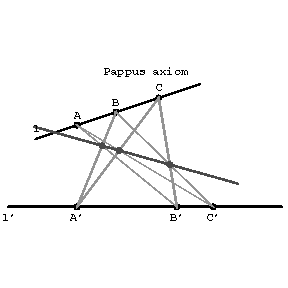Pappus axiom

If $l$ and $l'$ are two distinct straight lines and $A,B,C$ and $A',B',C'$ are distinct points on $l$ and $l'$, respectively, and if none of these is the point of intersection of $l$ and $l'$, then the points of intersection of $AB'$ and $A'B$, $BC'$ and $B'C$, $AC'$ and $A'C$ are collinear.